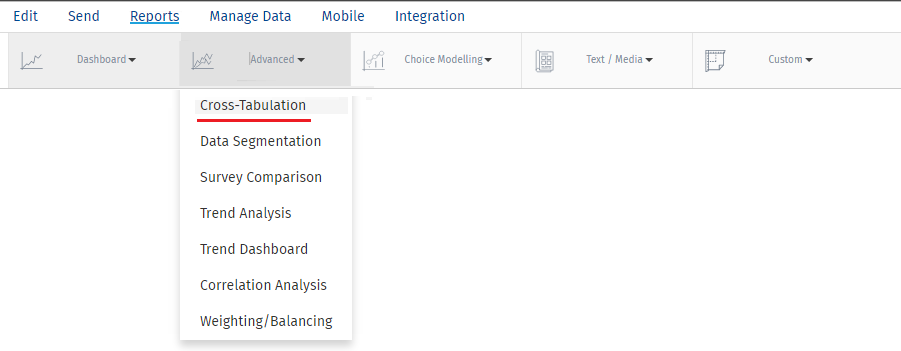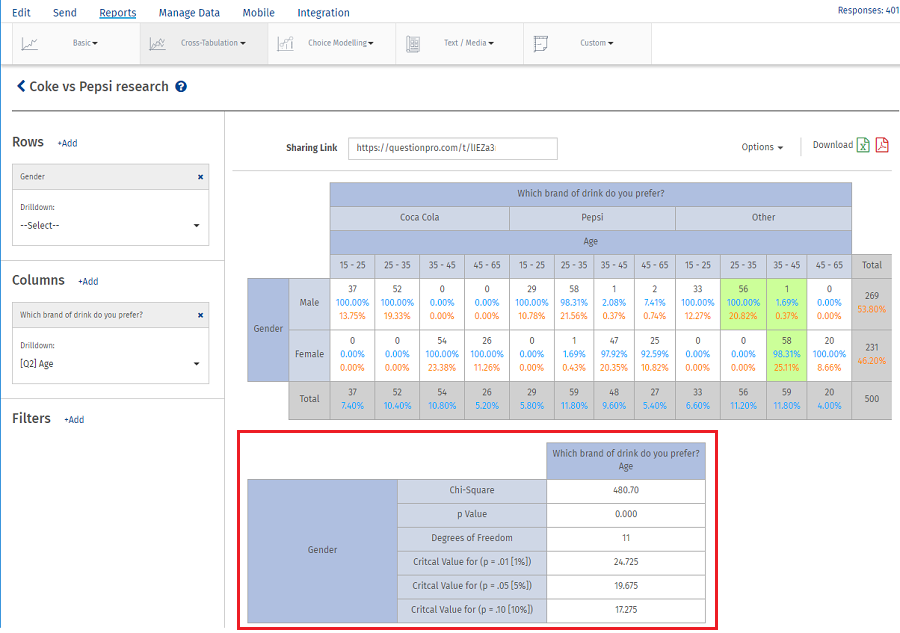# Cross Tabulation - Pearson’s Chi-Square Test

Chi-Square Test is used to see if there is an association between the categorical variables or not. We formulate two hypothesis.

Null Hypothesis: There is no significant relationship between variables.

Alternative Hypothesis: There is a significant relationship between variables.Chi-Square calculation is done using the above formula
O - Observed Frequency
E - Expected Frequency

Significance Levels α = 0.01, α = 0.05, α = 0.10

Chi-Square Critical value is obtained from Chi -table using Degree of freedom and Significance level α.

If the chi calculated > chi critical or p-value < 0.05 (decided significance level), we Reject the Null Hypothesis. We state there exist significant relationship between the variables.

In the above mentioned Example Chi Square Calculated value is 624.37.

Chi Square Critical Value at Alpha = 0.01, 0.05, 0.10 is less then Chi Square Calculated value.

Here Chi Calculated > Chi Critical. Hence we state there exist a significant relationship between variables.In the above example the Chi Calculated value > Chi Critical
480.70>24.725 at alpha = 0.01
480.70>19.675 at alpha = 0.05
480.70>17.275 at alpha = 0.10

Hence we reject the Null hypothesis and state that there exist significant relationship between all 3 variables(Gender, Age and Drink). As there is relationship between 3 variables we can go for cell comparison test which will highlight the cell.